### EURUSD : Elliott wave forecast for the period of 24.06.22 to 01.07.22

Consider taking short positions from corrections below the level of 1.0767 with a goal of 1.0200–1.00000 as the main scenario.

An alternative possibility is that the pair will continue to rise to the levels of 1.0937–1.1184 after breaking out and consolidating above the level of 1.0767.

Analysis: According to the daily chart, the final wave (Z) of wave B is forming as part of a bearish correction that is likely still developing as wave of bigger degree B. On the H4 chart, wave C of (Z) seems to be developing, with the fifth wave of smaller degree v of C forming inside. A local correction is complete as wave (iv) of wave (v) on the H1 chart, wave (iii) of wave (v) created, and wave (v) of wave (v) is forming inside wave (iv) of wave (v).If the assumption is accurate, the pair will keep falling until it reaches levels between 1.0200 and 1.0000. In this case, the level of 1.0767 is crucial. The pair will be able to continue increasing after it breaks out to levels between 1.0937 and 1.1184.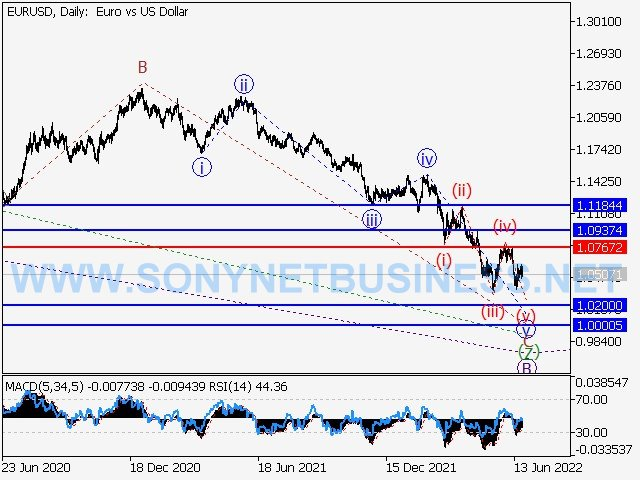EURUSD : Elliott wave forecast for the period of 24.06.22 to 01.07.22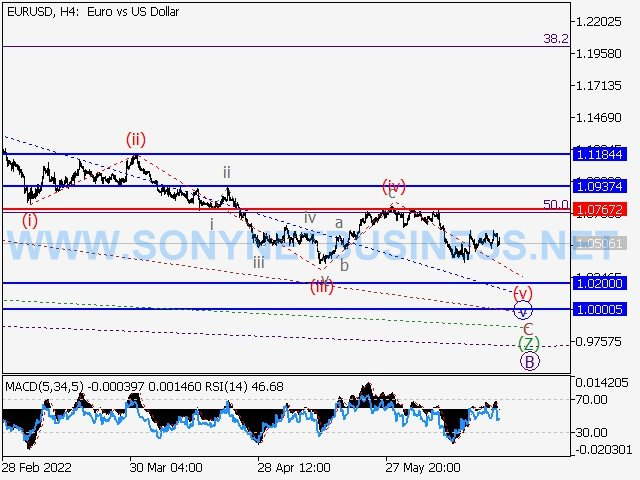EURUSD : Elliott wave forecast for the period of 24.06.22 to 01.07.22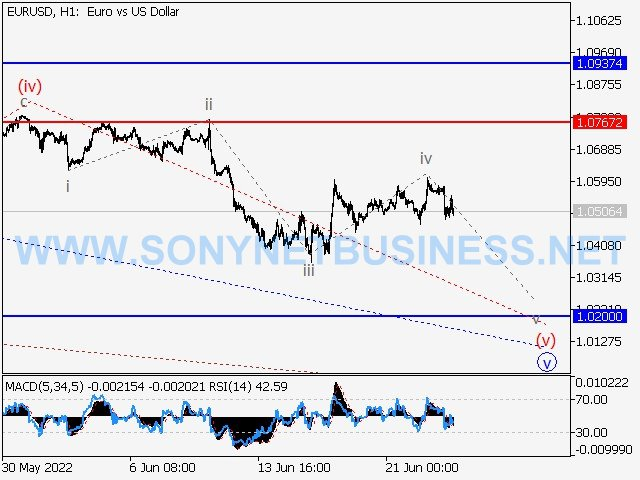EURUSD : Elliott wave forecast for the period of 24.06.22 to 01.07.22

### GBPUSD : Elliott wave forecast for the period of 24.06.22 to 01.07.22

The primary scenario is to think about taking short positions on dips below the level of 1.2400 with a goal of 1.1900 to 1.1730.

An alternative possibility is that the pair will continue to rise to levels between 1.3083 and 1.3302 after breaking out and consolidating above the level of 1.2400.

On the daily chart, the first wave of greater magnitude (1) forms, and a descending corrective continues to form as wave (2). (2). On the H4 chart, wave C of (2) is developing, with the fifth wave v of C forming as a part of it. On the H1 chart, wave iv of (v) and wave v of (v) are developing inside of a local correction as the fifth wave of smaller degree (v) of v looks to be forming. If the assumption is accurate, the pair will keep falling until it reaches values between 1.1900 and 1.1730. In this scenario, the level of 1.2400 is crucial since a breakout there would allow the pair to continue advancing to levels between 1.3083 and 1.3302.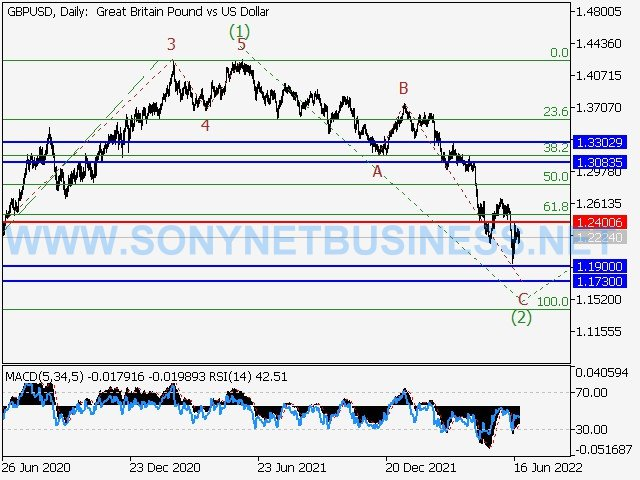GBPUSD : Elliott wave forecast for the period of 24.06.22 to 01.07.22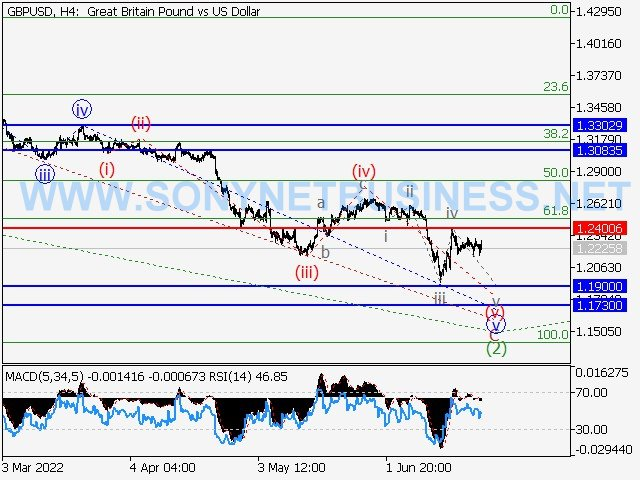GBPUSD : Elliott wave forecast for the period of 24.06.22 to 01.07.22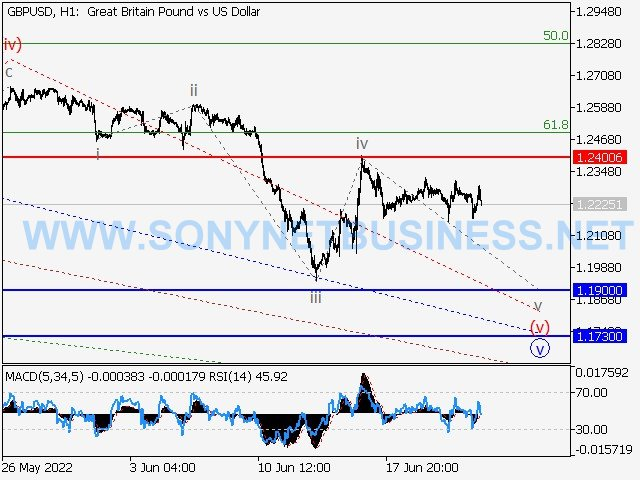GBPUSD : Elliott wave forecast for the period of 24.06.22 to 01.07.22

### USDJPY : Elliott wave forecast for the period of 24.06.22 to 01.07.22

Aim for long positions with a target of 140.00 to 145.00 from corrections above the level of 131.44.

An alternative scenario is that the pair will continue to decline to levels between 124.90 and 121.57 with a breakout and consolidation below the level of 131.44.

Analysis: Wave 3 of (3) is forming as the third wave of bigger degree (3), which is still developing on the daily chart. According to the H4 chart, the fifth wave, a smaller degree v of 3, is still emerging. On the H1 chart, wave (iii) of v looks to have formed, and wave (iv) of v is ending as a local correction. If the assumption is accurate, the pair will increase to between 140.00 and 145.00. In this scenario, the level of 131.44 is crucial since a breakout there would allow the pair to keep falling to the levels of 124.90 and 121.57.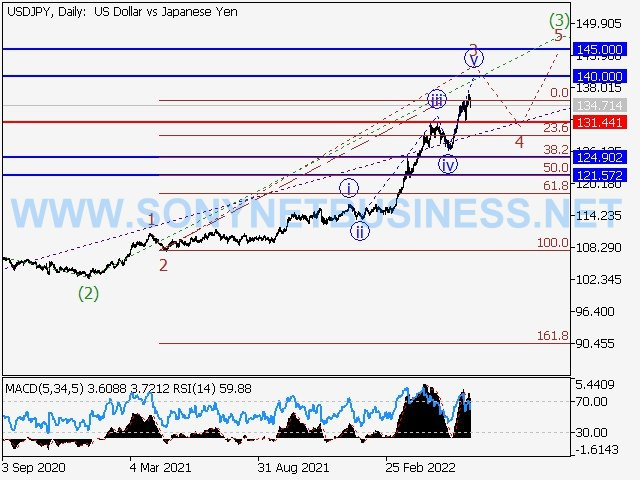USDJPY : Elliott wave forecast for the period of 24.06.22 to 01.07.22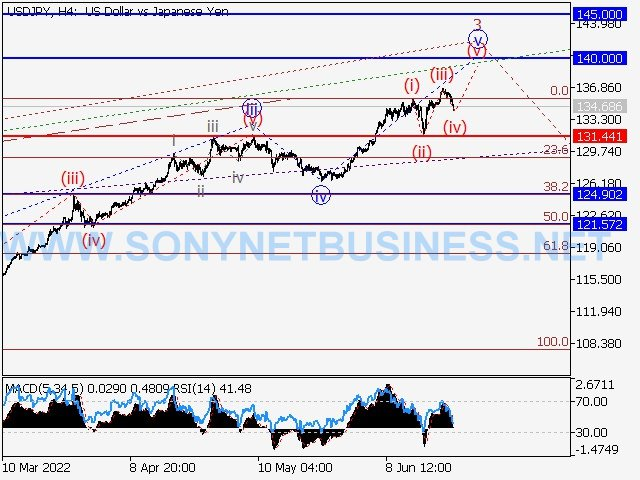USDJPY : Elliott wave forecast for the period of 24.06.22 to 01.07.22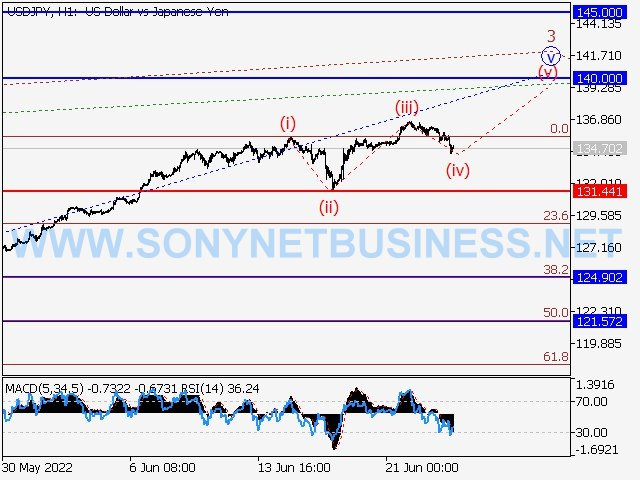USDJPY : Elliott wave forecast for the period of 24.06.22 to 01.07.22

### USDCHF : Elliott wave forecast for the period of 24.06.22 to 01.07.22

Main scenario: Once a correction emerged, think about taking long positions above the level of 0.9502 with a target of 1.0200–1.0400.

An alternative scenario is that the pair will continue to decline to levels between 0.9194 and 0.9088 if breakout and consolidation below 0.9502 occur.

Analysis: Wave 3 of (3) is emerging as the third wave of bigger degree (3) on the daily chart. On the H4 chart, a local correction is forming as the fourth wave of the third wave of smaller degree, which is wave iii of 3. On the H1 chart, wave (a) of iv appears to have ended. If the assumption is true, after the corrective wave iv of wave 3 is finished, the pair will continue to rise to the levels of 1.0200 - 1.0400. In this case, the level of 0.9502 is crucial. If it breaks out, the pair will be free to keep sliding until it reaches levels of 0.9194 and 0.9088.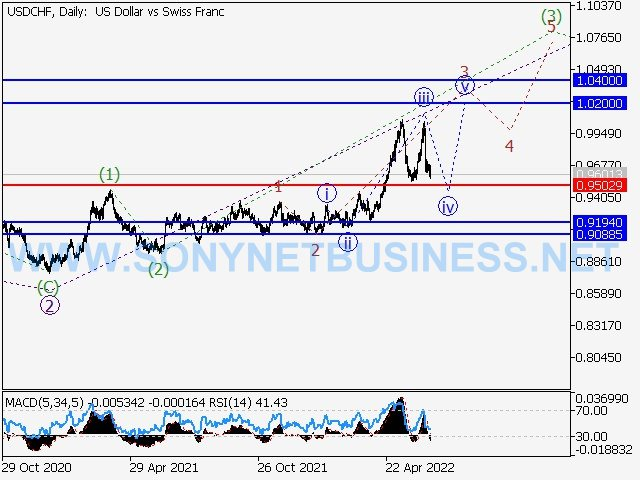USDCHF : Elliott wave forecast for the period of 24.06.22 to 01.07.22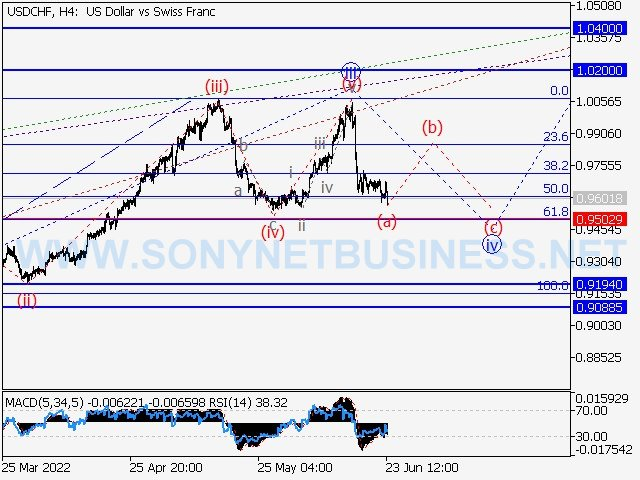USDCHF : Elliott wave forecast for the period of 24.06.22 to 01.07.22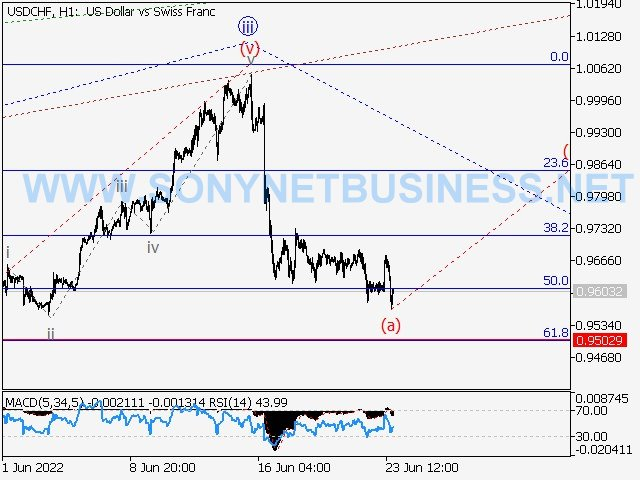USDCHF : Elliott wave forecast for the period of 24.06.22 to 01.07.22

### USDCAD : Elliott wave forecast for the period of 24.06.22 to 01.07.22

1.2410–1.2280 would be the target range for short positions to be taken from corrections below the level of 1.3075.

An alternative possibility is that the pair will continue to rise to levels between 1.3250 and 1.3431 after breaking out and consolidating above the level of 1.3075.

Analysis: On the daily chart, it appears that a descending corrective reached its conclusion as the larger fourth wave 4 and that the fifth wave 5 has begun to take shape. On the H4 chart, a local correction is forming as the second wave 2 of (1) of the first counter-trend wave of smaller degree 1 of (1) of 5. On the H1 chart, it appears that wave an of wave 2 formed, wave b of wave 2 finished developing, and wave c of wave 2 began forming. If the assumption is accurate, the pair will keep falling until it reaches values between 1.2410 and 1.2280. In this scenario, the level of 1.3075 is crucial since a breakout there would allow the pair to continue advancing to levels between 1.3250 and 1.3431.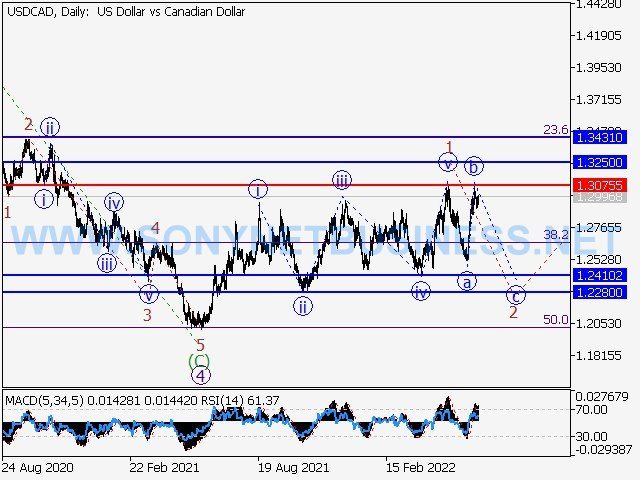USDCAD : Elliott wave forecast for the period of 24.06.22 to 01.07.22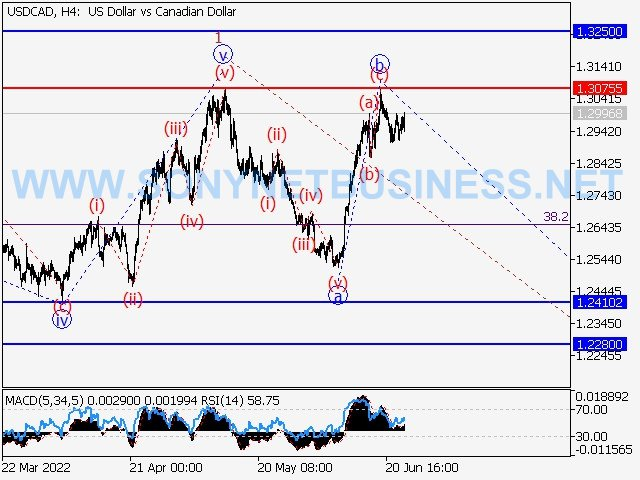USDCAD : Elliott wave forecast for the period of 24.06.22 to 01.07.22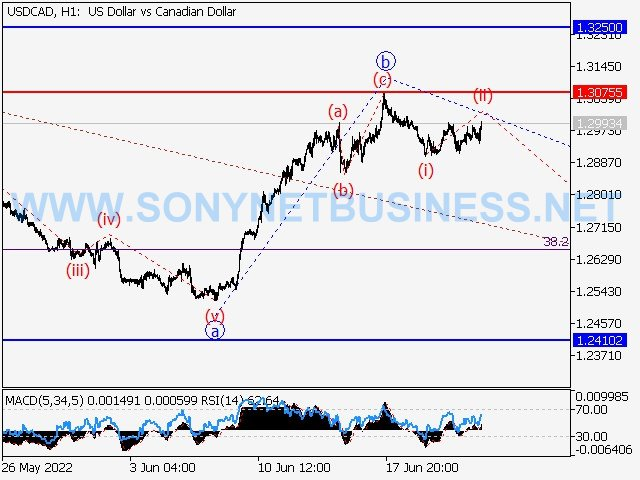USDCAD : Elliott wave forecast for the period of 24.06.22 to 01.07.22

### WTI Crude Oil : Elliott wave forecast for the period of 24.06.22 to 01.07.22

The primary scenario is to think about taking short positions from corrections below the level of 116.32 with a target range of 93.70 to 84.25.

Alternative scenario: The asset will continue to rise to the levels of 123.95 - 132.00 with a breakout and consolidation above the level of 116.32.

Analysis: based on the daily chart, it appears like wave 3 of the bigger degree initial wave (1) is still forming. On the H4 chart, the fourth wave of (1), which is most likely a local correction, is developing as wave c of 4. On the H1 chart, wave I of c appears to be finished. After wave (ii) of c has finished, if the assumption is accurate, the asset's price will continue to decrease until it reaches the range of 93.70 - 84.25. In this scenario, the level of 116.32 is crucial since a breach there would allow for further price growth to levels between 123.95 and 132.00.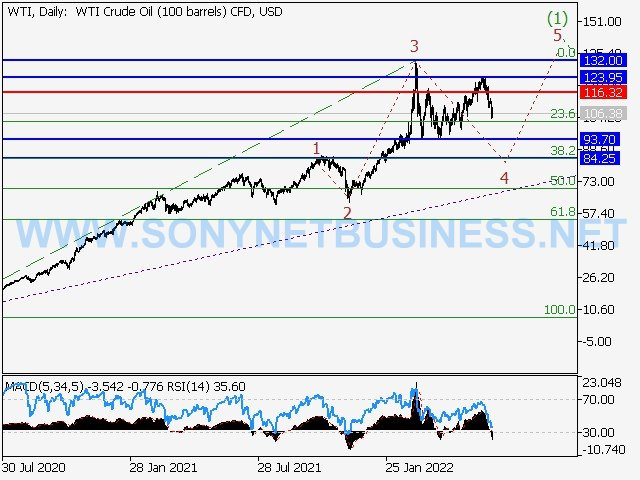WTI Crude Oil : Elliott wave forecast for the period of 24.06.22 to 01.07.22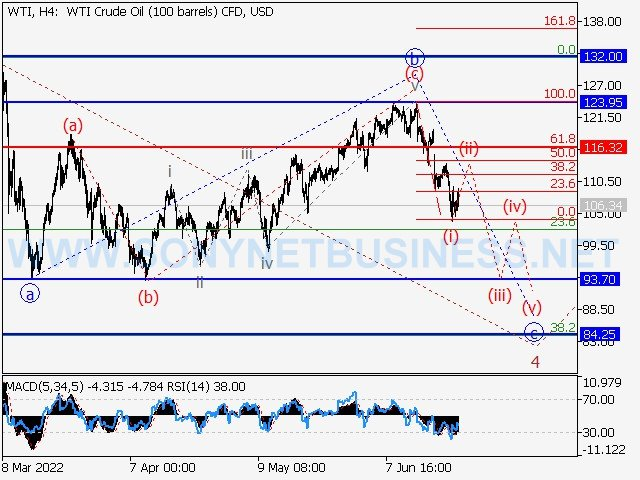WTI Crude Oil : Elliott wave forecast for the period of 24.06.22 to 01.07.22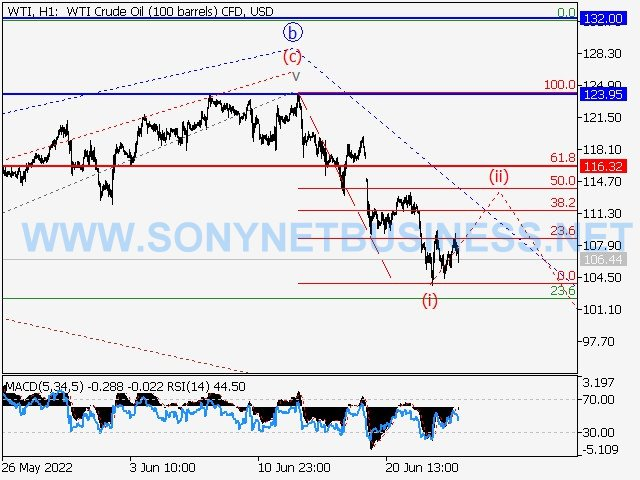WTI Crude Oil : Elliott wave forecast for the period of 24.06.22 to 01.07.22

### GOLD : Elliott wave forecast for the period of 24.06.22 to 01.07.22

The primary scenario is to think about taking long positions on corrections over the level of 1805.08 with a target of 1997.97 to 2070.98.

An alternative scenario is that the pair will continue to decline to levels between 1722.24 and 1616.95 if breakout and consolidation below the level of 1805.08 occur.

Analysis: The fifth wave 5 of the greater degree (3) is building inside the third wave of increasing degree (3), which is still developing on the daily chart. The fourth wave of wave iv of wave 5 on the H4 chart developed after wave iii of wave 5 appeared to have finished.On the H1 chart, the first counter-trend wave of smaller degree I of v formed, a local correction (ii) of v was finished, and wave (iii) of v was developing inside. This suggests that the fifth wave v of 5 has begun to develop. The pair will increase further to the levels of 1997.97 - 2070.98 if the assumption is right. In this situation, the level of 1805.08 is crucial because a breach of it would allow the pair to continue falling to the levels of 1722.24 and 1616.95.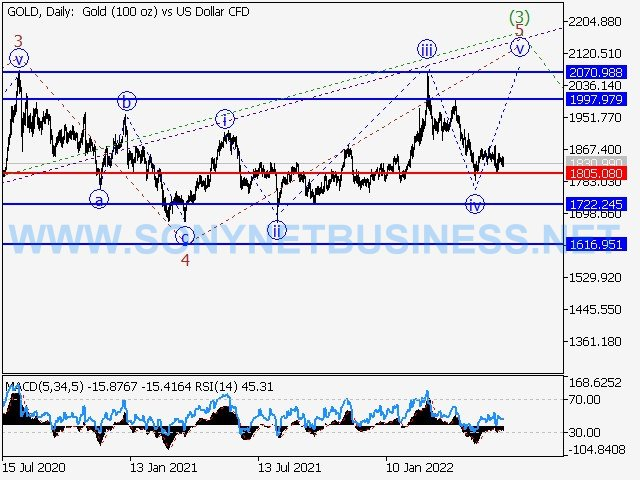GOLD : Elliott wave forecast for the period of 24.06.22 to 01.07.22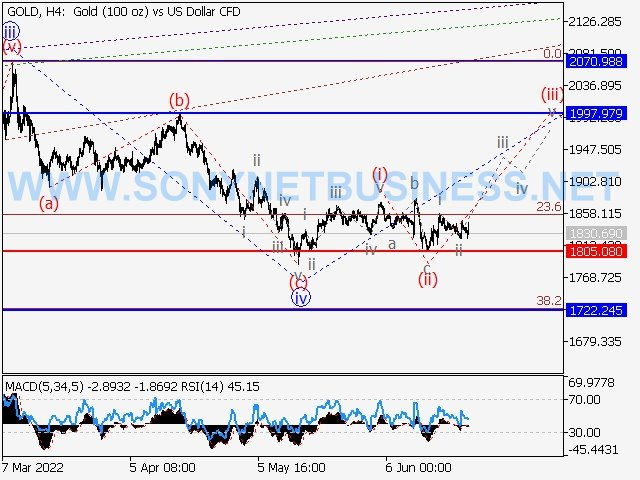GOLD : Elliott wave forecast for the period of 24.06.22 to 01.07.22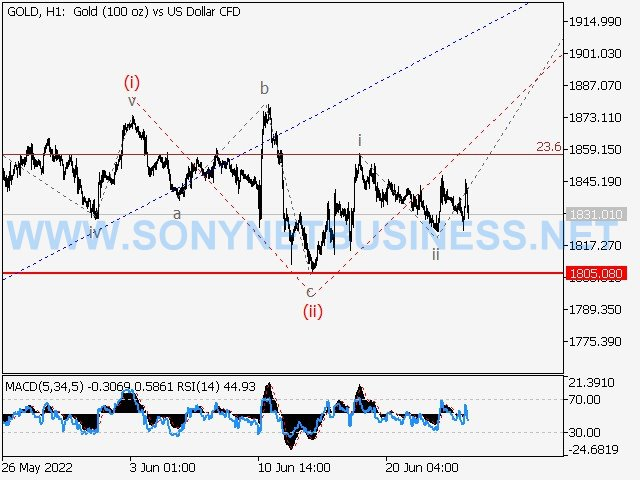GOLD : Elliott wave forecast for the period of 24.06.22 to 01.07.22

The Analytical Materials are Provided by, SONY Net Business A Trader and Analyst.